Courses

# Test: Simple Trusses

## 30 Questions MCQ Test Engineering Mechanics | Test: Simple Trusses

Description
This mock test of Test: Simple Trusses for Mechanical Engineering helps you for every Mechanical Engineering entrance exam. This contains 30 Multiple Choice Questions for Mechanical Engineering Test: Simple Trusses (mcq) to study with solutions a complete question bank. The solved questions answers in this Test: Simple Trusses quiz give you a good mix of easy questions and tough questions. Mechanical Engineering students definitely take this Test: Simple Trusses exercise for a better result in the exam. You can find other Test: Simple Trusses extra questions, long questions & short questions for Mechanical Engineering on EduRev as well by searching above.
QUESTION: 1

### __________ is a structure made of slender members which are joined together at their end points.

Solution:

The truss is a structure made of slender members which are joined together at their end points. They can be of wooden or steel. But most probably they are made from stainless steel. As they need to support the loadings in various climates.

QUESTION: 2

### ________ trusses lie on a plane.

Solution:

The planar trusses lie on a plane. Like for e.g. the trusses in the bridges. These trusses are the main supports of the bridge. They are extended straight vertical and are strong enough to resist various changes in the weather.

QUESTION: 3

### In a roof supporting truss the load is transmitted when ________

Solution:

The roof load is transmitted to the truss first. It is then transmitted to the joints. This transmission of the force or the load from trusses to the joints is achieved by the purlins. Thus the distribution of the load.

QUESTION: 4

As the loading is acting in the two dimensions, that is in a single plane. Thus the calculations involved in the trusses are in 2D.

Solution:

The loading is acting in a plane. Thus the calculations are done in 2D only. As the equations for 3D are different. Although the use of vectors can make our task easy but still 2D calculations are done for the trusses, as they are acting in a same plane.

QUESTION: 5

In case of bridge the load is transferred when __________

Solution:

The bridge load is transmitted to the stringers first. It is then transmitted to the floor beams. The load from beams is then transmitted to the joints. Thus the transmission of the load of the bridge.

QUESTION: 6

As the loading in bridge different from the simple trusses the calculations involved in the bridges are all 2D calculations.

Solution:

The loading in the bridge is acting in a plane. Thus the calculations are done in 2D only. As the equations for 3D are different. Although the use of vectors can make our task easy but still 2D calculations are done for the trusses, as they are acting in a same plane. But the thing is that the loading in bridge and trusses are same.

QUESTION: 7

When the bridges are extended over long routes or distance then _________

Solution:

When the bridges are extended over long routes or distance then a rocker or a roller is used at the joints. This allows the bridge joints to move around. That is when the temperature is raised. The elongations and the contractions of the joints part are not much affected if the rollers and rockers are used.

QUESTION: 8

Find the force in the member RP of the frame shown below.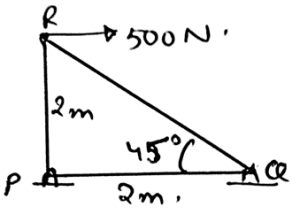Solution:

The direction of the unknown is not known to us. To know the direction of the unknown force we take assumption of it. That is we assume that the particular direction might be the direction of the force and then we do the calculations accordingly. And then we apply the equilibrium equations to the joints.

QUESTION: 9

To design the trusses which of the following rules is followed?

Solution:

The set of rules which are used to design the trusses are having various rules. To them one is that the loads are applied at the joints. This is done with the neglecting of the weight of the trusses section.

QUESTION: 10

The rules which are used to design the trusses are having various rules. Of them one is that the smooth pins are not used to join the members.

Solution:

The set of rules used to design the trusses are having various rules. Of them one is that the smooth pins are used to join the members. The joint are generally formed by welding the materials at the ends of the trusses. Which gives strength to the design.

QUESTION: 11

Find the force in the member RQ of the frame shown below.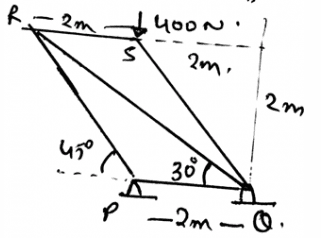Solution:

The direction of the unknown is not known to us. To know the direction of the unknown force we take assumption of it. That is we assume that the particular direction might be the direction of the force and then we do the calculations accordingly. And then we apply the equilibrium equations to the joints.

QUESTION: 12

Find the force in the member QS of the frame shown below.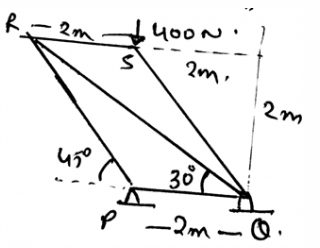Solution:

The direction of the unknown is not known to us. To know the direction of the unknown force we take assumption of it. That is we assume that the particular direction might be the direction of the force and then we do the calculations accordingly. And then we apply the equilibrium equations to the joints.

QUESTION: 13

A _________ truss is in triangular section.

Solution:

A simple truss in the shape of a triangle. It is the equilateral triangle. That is the angle between the legs are 60. This means the load is divided according and are equal in all the legs of the truss. Hence the simple truss.

QUESTION: 14

Find the force in the member PQ of the frame shown below.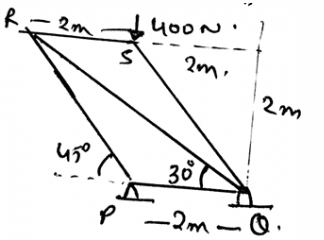Solution:

The direction of the unknown is not known to us. To know the direction of the unknown force we take assumption of it. That is we assume that the particular direction might be the direction of the force and then we do the calculations accordingly. And then we apply the equilibrium equations to the joints.

QUESTION: 15

The joint in the trusses are generally formed by welding the materials at the ends of the trusses on a common plate. Which gives strength to the design. What is that plate called?

Solution:

The joint in the trusses are generally formed by welding the materials at the ends of the trusses on a common plate. Which gives strength to the design. This plate is known as Gusset plate. This plate holds up various trusses that are being welded to it , to make the structure strong.

QUESTION: 16

Which of the following is correct?

Solution:

The direction of the unknown is not known to us. To know the direction of the unknown force we take assumption of it. That is we assume that the particular direction might be the direction of the force and then we do the calculations accordingly.

QUESTION: 17

Find the force in the member RQ of the frame shown below.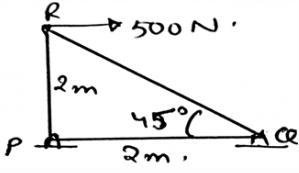Solution:

The direction of the unknown is not known to us. To know the direction of the unknown force we take assumption of it. That is we assume that the particular direction might be the direction of the force and then we do the calculations accordingly. And then we apply the equilibrium equations to the joints.

QUESTION: 18

Trusses lie in a single plane. And the calculations used to solve the forces are from vector math.

Solution:

The loading is acting in a plane. Thus the calculations are done in 2D only. As the equations for 3D are different. Although the use of vectors can make our task easy but still 2D calculations are done for the trusses, as they are acting in a same plane.

QUESTION: 19

The transmission of the load on a certain type of a structure follow as from stringers to floor beams and then joints. Determine the structure.

Solution:

The transmission of the load on the bridges follow as from stringers to floor beams and then joints. This is because of the first the stringers come in contact with the force. Which gets distributed to them. Then comes the floor beams, which takes that distributed loadings.

QUESTION: 20

When the bridges are extended over long routes or distance then a rocker or a roller is used at the joints. As when the temperature is raised the elongations and the contractions of the joints part are not much affected if the rollers and rockers are used.

Solution:

The roller and the rockers take over the elongations and the contractions of the joints part. And thus help in stabilising the structure. Thus when the bridges are extended over long routes a rocker or a roller is used at the joints.

QUESTION: 21

Find the force in the member RS of the frame shown below.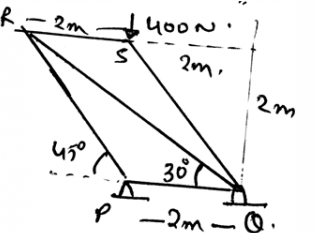Solution:

The direction of the unknown is not known to us. To know the direction of the unknown force we take assumption of it. That is we assume that the particular direction might be the direction of the force and then we do the calculations accordingly. And then we apply the equilibrium equations to the joints.

QUESTION: 22

There are no rules for the designing of the truss. But there are rules designing the bridges.

Solution:

There are various rules used to design the truss. Like the loads must be at the joints only. The use of pin must be there in the joining of the trusses etc. Also the joints must be welded with a gusset plate. And the bridges are also having various rules for their design too.

QUESTION: 23

A truss is facing a two force member and both of them are in the opposite directions. And the truss is in equilibrium. And both of them are getting away from each other.The forces are called?

Solution:

If two forces are in the opposite directions. And are collinear too. Then the truss is getting a tensile stress. As the forces are trying to increase the length of the truss. Hence if the two force members are in such conditions the forces are tensile forces.

QUESTION: 24

A truss is facing a two force member and both of them are in the opposite directions. And the truss is in equilibrium. And both of them are coming towards each other. The forces are called __________

Solution:

If two forces are in the opposite directions. And both of them are coming towards each other. And are collinear too. Then the truss is getting a compressive stress. As the forces are trying to decrease the length of the truss. Hence if the two force members are in such conditions the forces are compressive forces.

QUESTION: 25

Find the force in the member PR of the frame shown below.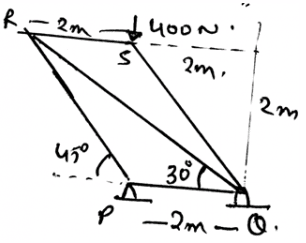Solution:

The direction of the unknown is not known to us. To know the direction of the unknown force we take assumption of it. That is we assume that the particular direction might be the direction of the force and then we do the calculations accordingly. And then we apply the equilibrium equations to the joints.

QUESTION: 26

Truss cannot be acted upon three force members. Because the truss in a single plane.

Solution:

The singular plane of the truss doesn’t mean that the trusses cannot be acted upon the three force system. They can be acted upon the three force system, possibility is that the forces might not be collinear or might be parallel to each other. But point is the trusses can be acted on the three force members too, still conditions need to specific.

QUESTION: 27

Find the force in the member QP of the frame shown below.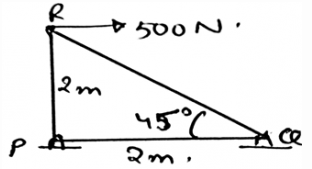Solution:

The direction of the unknown is not known to us. To know the direction of the unknown force we take assumption of it. That is we assume that the particular direction might be the direction of the force and then we do the calculations accordingly. And then we apply the equilibrium equations to the joints.

QUESTION: 28

Which of the following is true?

Solution:

We can take such assumptions. We are free to do so. We just need to specify if clockwise is positive then the counter clockwise is to be negative. Also such assumption are very useful in 2D calculation of the moments caused by various forces on the body.

QUESTION: 29

The moment is the cross product of which two vectors?

Solution:

The cross product needs to take in the proper sequence. If not taken then the answer is just the opposite of the true answer. That’s why, the answer is not the Force and Radius vectors, but the Radius and Force vectors. Because the moment has its direction, as many of the cross products have, and thus precaution needs to be taken.

QUESTION: 30

The ___________ forces do not cause the rotation.

Solution:

The concurrent forces are the which are somewhere touching the axis of rotation. If any of the force is touching that axis, that force is not considered, or is insufficient to cause a rotation. If a force is concurrent then the perpendicular distance of the force from the line of axis is zero, thus no rotation. As we know rotation is caused by moment.